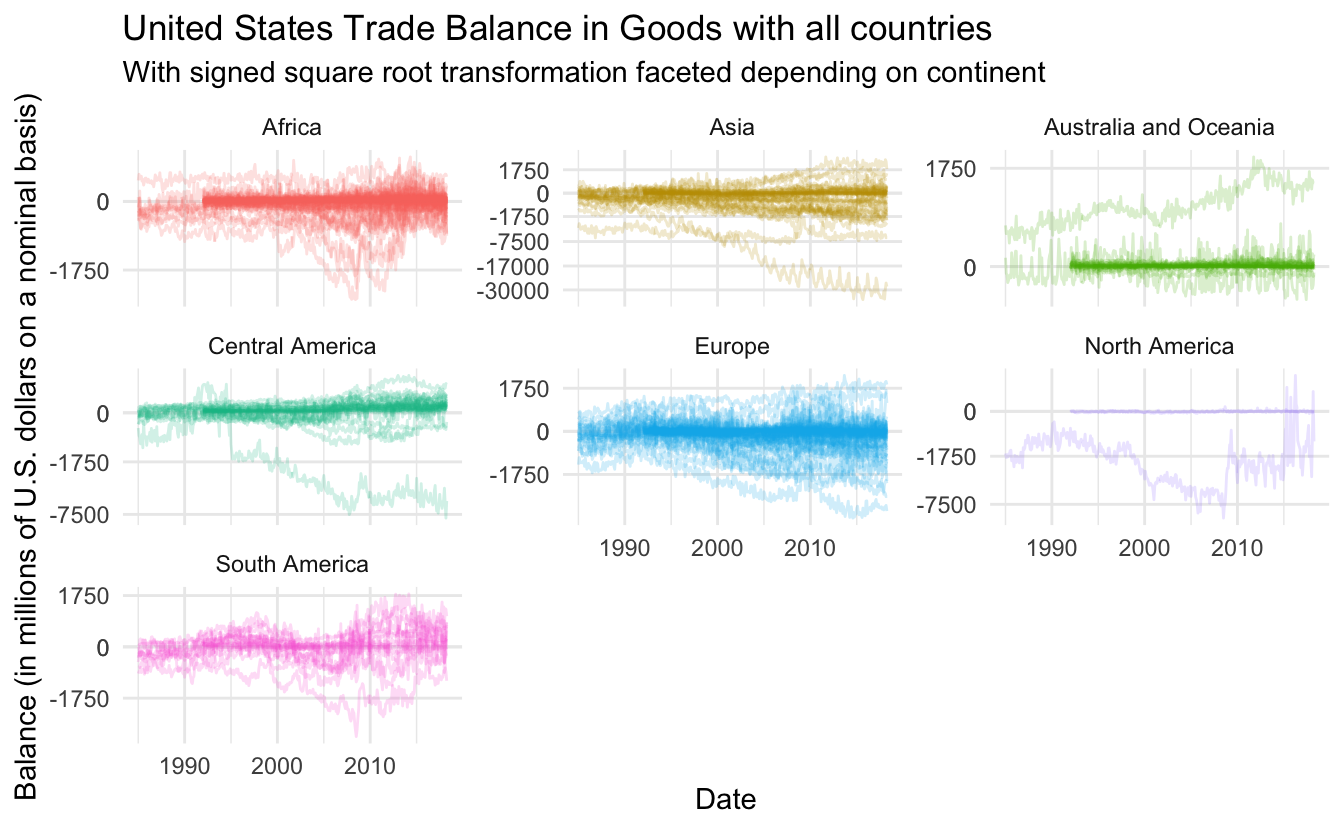# ggplot2 trial and error - US trade data

This code have been lightly revised to make sure it works as of 2018-12-16.

This blogpost will showcase an example of a workflow and its associated thought process when iterating though visualization styles working with `ggplot2`. For this reason will this post include a lot of sub par charts as you are seeing the steps not just the final product.

We will use census data concerning US trade with other nations which we scrape as well.

## Setting up

We will using a standard set of packages, `tidyverse` for general data manipulation, `rvest` and `httr` for scraping and manipulation.

``````library(tidyverse)
library(rvest)
library(httr)``````

## Getting the data

This project started when I found the following link https://www.census.gov/foreign-trade/balance/c4099.html. It include a month by month breakdown of U.S. trade in goods with Denmark from 1985 till the present. Unfortunately the data is given in yearly tables, so we have a little bit of munching to do. First we notice that the last part of the url include `c4099`, which after some googling reveals that 4099 is the country code for Denmark. The fill list of country trade codes are given on the following page https://www.census.gov/foreign-trade/schedules/c/countrycode.html which also include a `.txt` file so we don’t have to scrape. We will remove the first entry and last 6 since US doesn’t trade with itself.

``````continent_df <- tibble(number = as.character(1:7),
continent = c("North America", "Central America",
"South America", "Europe", "Asia",
"Australia and Oceania", "Africa"))

skip = 5) %>%
tibble(code = .) %>%
separate(code, c("code", "name", "iso"), sep = "\\|") %>%
mutate_all(trimws) %>%
mutate(con_code = str_sub(code, 1, 1)) %>%
filter(!is.na(iso),
name != "United States of America",
con_code != 9) %>%
left_join(continent_df, by = c("con_code" = "number")) %>%
select(-con_code)``````

With these code we create the targeted urls we will be scraping

``target_urls <- str_glue("https://www.census.gov/foreign-trade/balance/c{code_df\$code}.html")``

We will be replication hrbrmstr’s scraping code found here since it works wonderfully.

``````s_GET <- safely(GET)

pb <- progress_estimated(length(target_urls))
map(target_urls, ~{
pb\$tick()\$print()
Sys.sleep(5)
s_GET(.x)
}) -> httr_raw_responses

good_responses <- keep(httr_raw_responses, ~!is.null(.x\$result))``````

then we wrangle all the html files by extracting all the tables, parse the numeric variables and combining them to one table.

``````wrangle <- function(x, name) {
# Read html and extract tables
html_nodes("table") %>%
html_table() %>%
# parse numeric columns
map(~ mutate_at(.x, vars(Exports:Balance), funs(parse_number))) %>%
bind_rows() %>%
mutate(Country = name)
}

full_data <- map2_df(good_responses, code_df\$code, wrangle)``````

Lastly we do some polishing up with the date variables and join in the country information.

``````trade_data <- full_data %>%
filter(!str_detect(Month, "TOTAL")) %>%
mutate(Date = parse_date(Month, format = "%B %Y"),
Month = lubridate::month(Date),
Year = lubridate::year(Date)) %>%
left_join(code_df, by = c("Country" = "code"))``````

Giving us this data to work with:

``glimpse(trade_data)``
``````## Observations: 75,379
## Variables: 10
## \$ Month     <dbl> 1, 2, 3, 4, 1, 2, 3, 4, 5, 6, 7, 8, 9, 10, 11, 12, 1...
## \$ Exports   <dbl> 0.1, 0.4, 1.8, 0.2, 0.1, 1.3, 3.2, 0.6, 0.3, 0.6, 0....
## \$ Imports   <dbl> 1.0, 2.4, 2.2, 0.8, 0.2, 0.5, 0.8, 0.9, 0.4, 2.4, 0....
## \$ Balance   <dbl> -0.8, -2.0, -0.4, -0.6, -0.1, 0.7, 2.4, -0.3, -0.1, ...
## \$ Country   <dbl> 1010, 1010, 1010, 1010, 1010, 1010, 1010, 1010, 1010...
## \$ Date      <date> 2018-01-01, 2018-02-01, 2018-03-01, 2018-04-01, 201...
## \$ Year      <dbl> 2018, 2018, 2018, 2018, 2017, 2017, 2017, 2017, 2017...
## \$ name      <chr> "Greenland", "Greenland", "Greenland", "Greenland", ...
## \$ iso       <chr> "GL", "GL", "GL", "GL", "GL", "GL", "GL", "GL", "GL"...
## \$ continent <chr> "North America", "North America", "North America", "...``````

## Lets get vizualising!

Lets set a different theme for now.

``theme_set(theme_minimal())``

Lets start out nice and simple by plotting a simple scatter plot for just a single country to get a feel for the data.

``````trade_data %>%
filter(name == "Greenland") %>%
ggplot(aes(Date, Balance)) +
geom_point() +
labs(title = "United States Trade Balance in Goods with Greenland")``````Which looks good already! Lets see how it would look with as a line chart instead

``````trade_data %>%
filter(name == "Greenland") %>%
ggplot(aes(Date, Balance)) +
geom_line() +
labs(title = "United States Trade Balance in Goods with Greenland")``````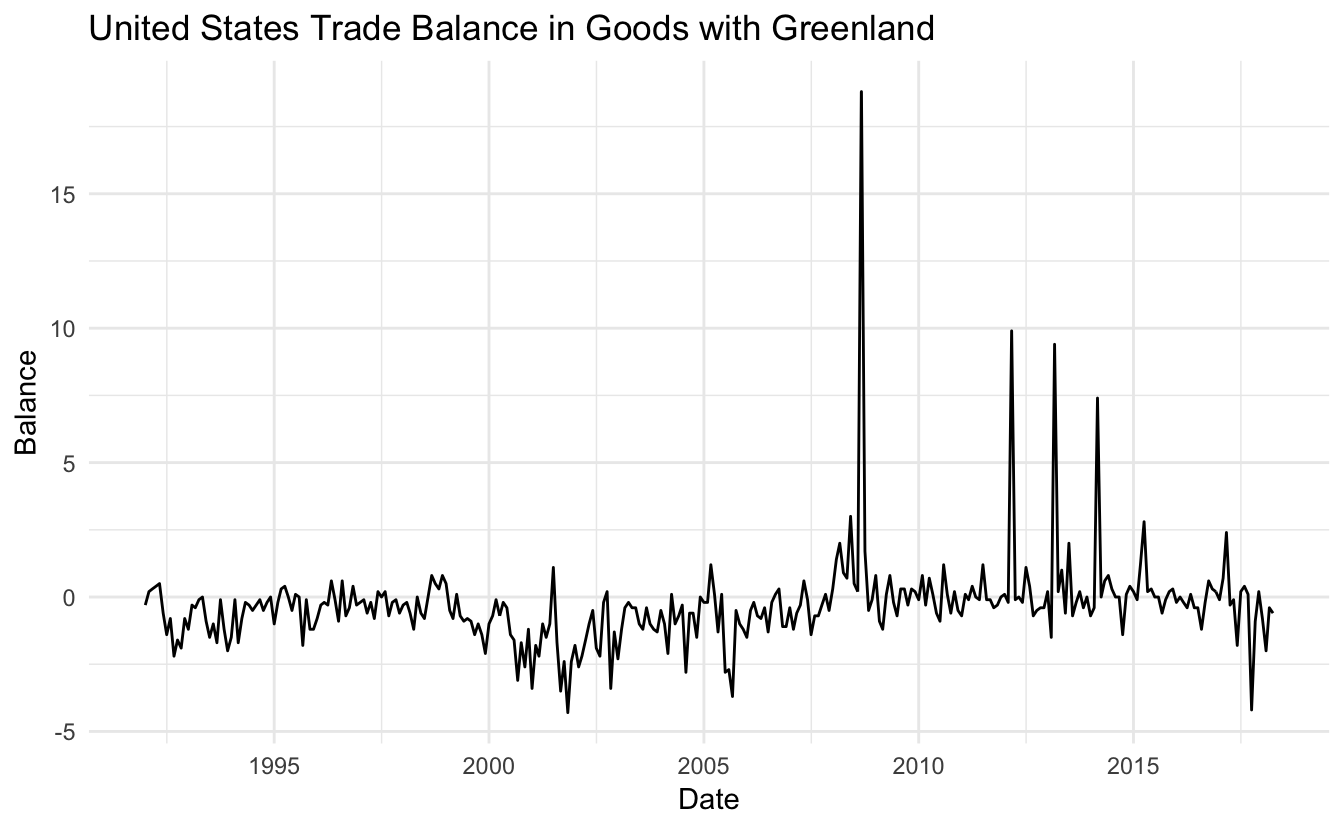it sure it quite jagged! Lets take a loot at the 4 biggest spiked to see if it is a indication of a trend

``````trade_data %>%
filter(name == "Greenland", Balance > 5)``````
``````## # A tibble: 4 x 10
##   Month Exports Imports Balance Country Date        Year name  iso
##   <dbl>   <dbl>   <dbl>   <dbl>   <dbl> <date>     <dbl> <chr> <chr>
## 1     3     7.9     0.5     7.4    1010 2014-03-01  2014 Gree… GL
## 2     3    10.4     1       9.4    1010 2013-03-01  2013 Gree… GL
## 3     3    10.5     0.6     9.9    1010 2012-03-01  2012 Gree… GL
## 4     9    20       1.3    18.8    1010 2008-09-01  2008 Gree… GL
## # ... with 1 more variable: continent <chr>``````

Which didn’t give us much, 3 of the spikes happened in March and the last one a random September. It was worth a try, back to plotting! Lets see how a smooth curve would look overlaid the line chart

``````trade_data %>%
filter(name == "Greenland") %>%
ggplot(aes(Date, Balance)) +
geom_line() +
geom_smooth(se = FALSE) +
labs(title = "United States Trade Balance in Goods with Greenland")``````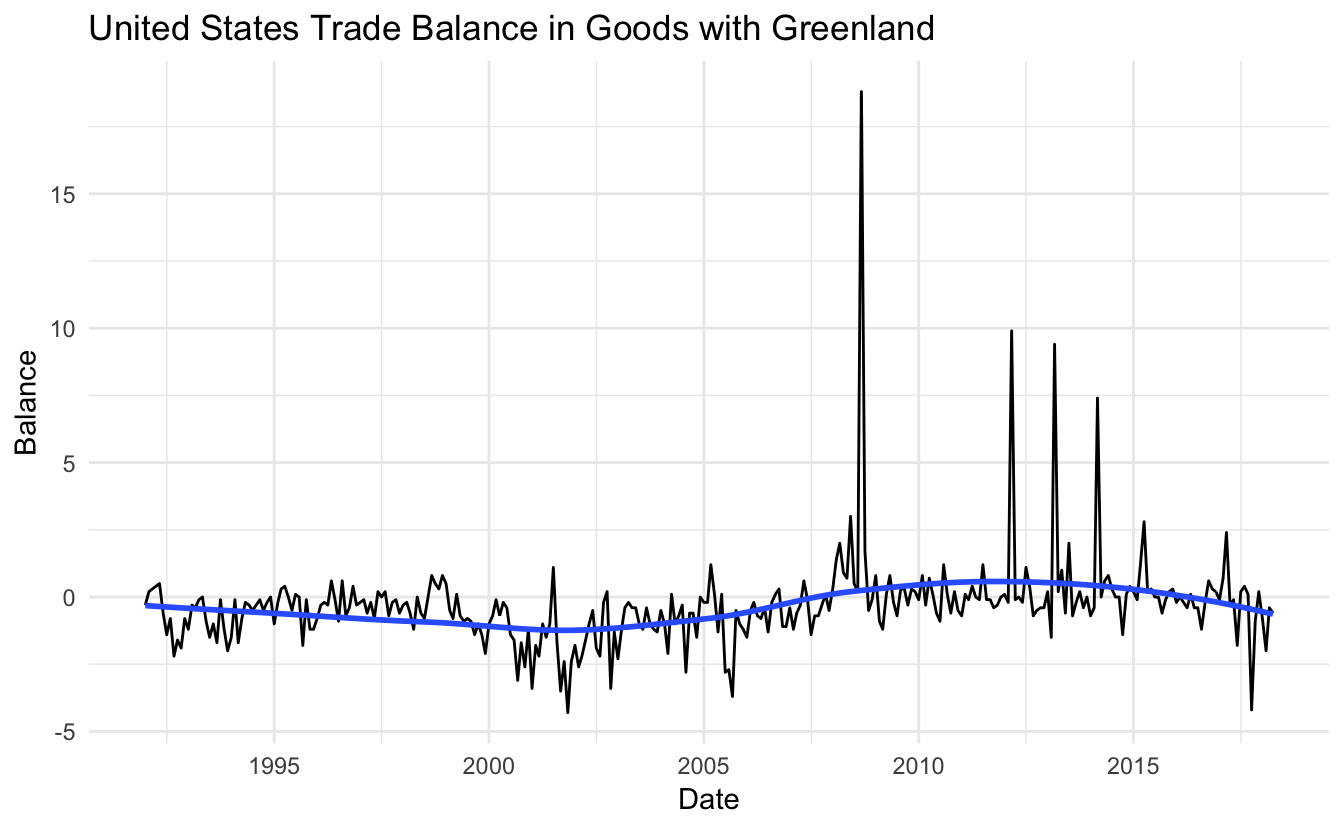Which looks nice in and off itself, however since this chart looks at the trade balance between two countries is the value 0 quite important and should be highlighted better. I will add a line behind the data points such that it highlights rather then hides

``````trade_data %>%
filter(name == "Greenland") %>%
ggplot(aes(Date, Balance)) +
geom_abline(slope = 0, intercept = 0, color = "orange") +
geom_line() +
geom_smooth(se = FALSE) +
labs(title = "United States Trade Balance in Goods with Greenland")``````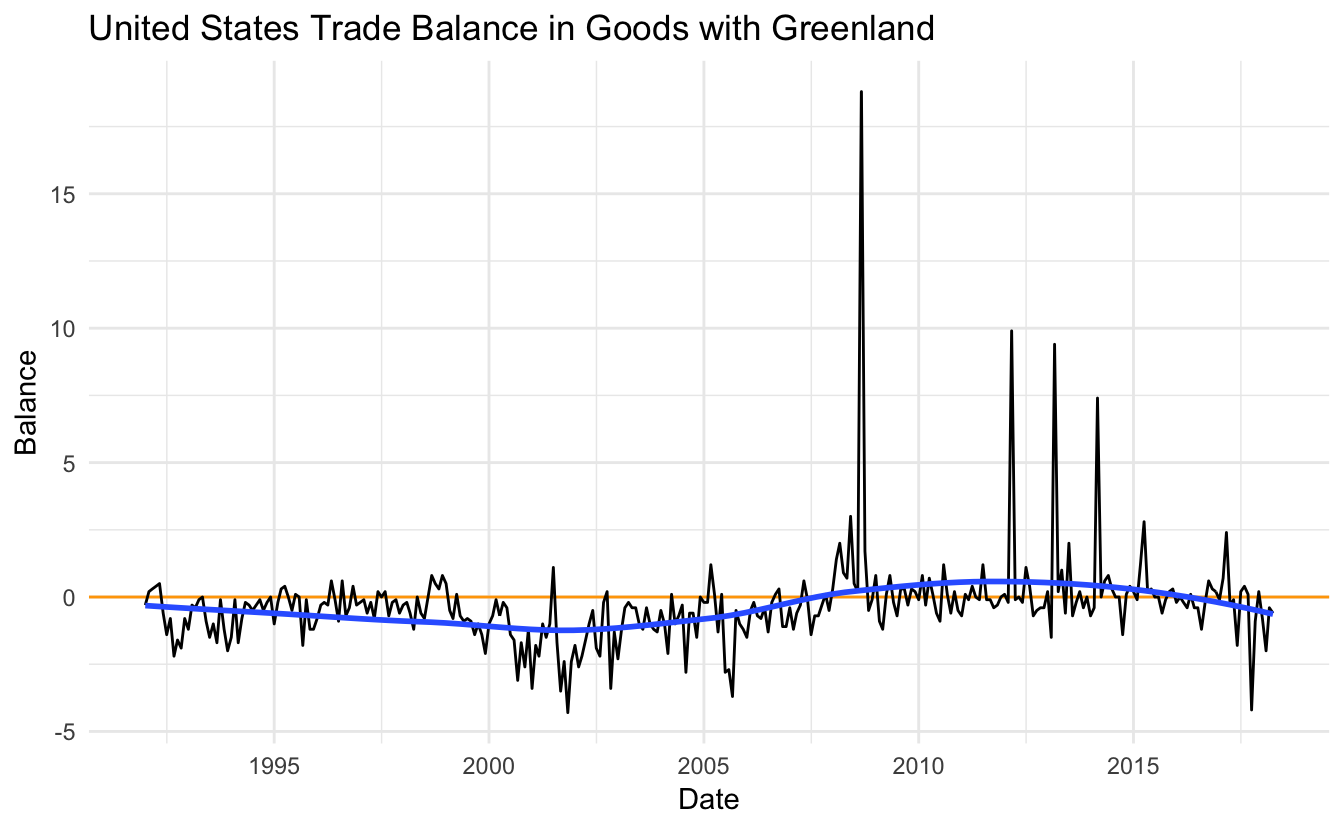This gives us better indication for when the trade is positive or negative with respect to the United States. Lets take it up a notch and include a couple more countries. We remove the filter and add `Country` as the `group` aesthetic.

``````trade_data %>%
ggplot(aes(Date, Balance, group = Country)) +
geom_line() +
labs(title = "United States Trade Balance in Goods with all countries")``````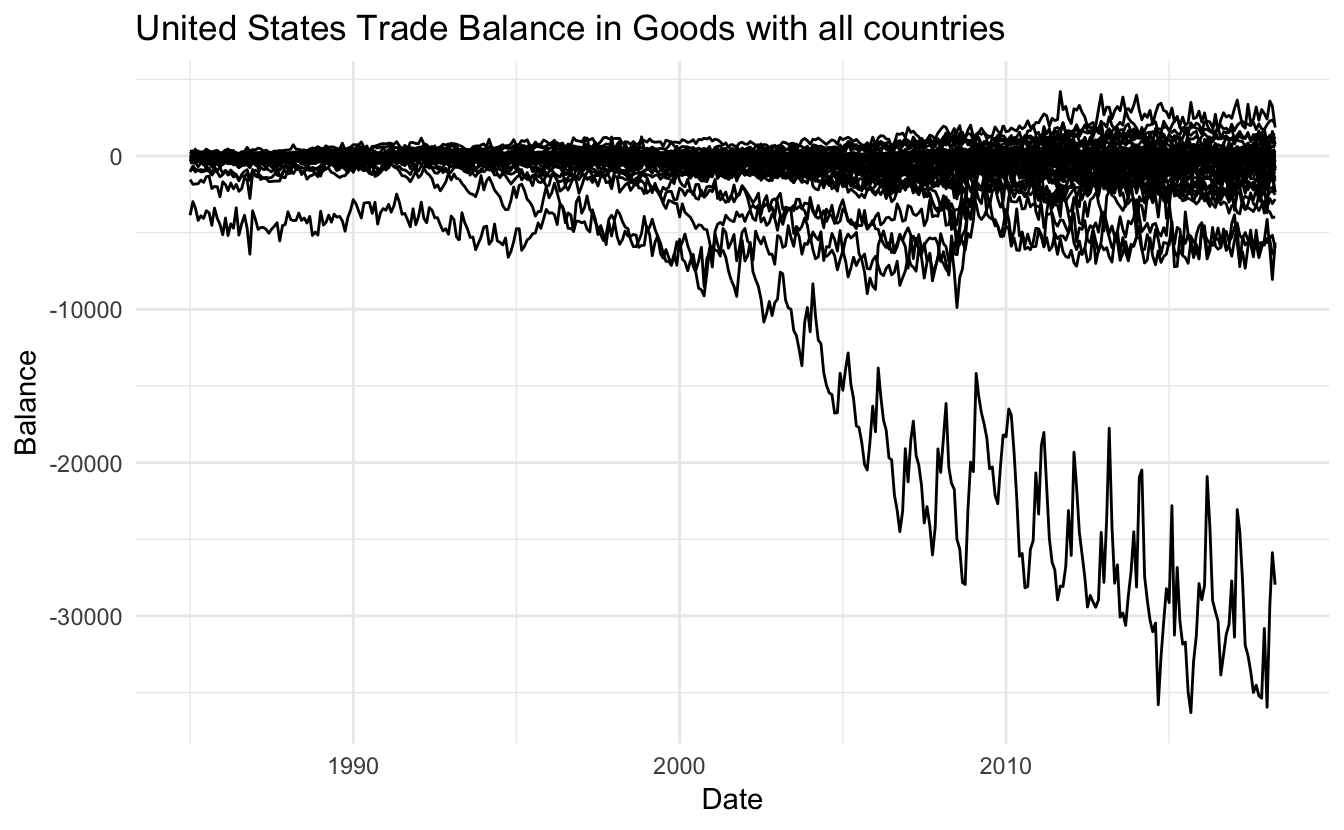So we have 3 different problems I would like to fix right now. The scale between these different countries is massively different! The very negative balance of one country is making it hard to see what happens to the other countries. Secondly it is hard to distinguish the different countries since they are all the same color. And lastly there is some serious over plotting, this point is tired to the other problems so lets see if we can fix them one at a time.

First lets transform the scales on the y axis such that we better can identify individual lines. We do this with the square root transformation which gives weights to values close to 0 and shrinks values far away form 0.

``````trade_data %>%
ggplot(aes(Date, Balance, group = Country)) +
geom_line() +
scale_y_sqrt() +
labs(title = "United States Trade Balance in Goods with all countries",
subtitle = "With square root transformation")``````
``## Warning in self\$trans\$transform(x): NaNs produced``
``## Warning: Transformation introduced infinite values in continuous y-axis``
``## Warning: Removed 11918 rows containing missing values (geom_path).``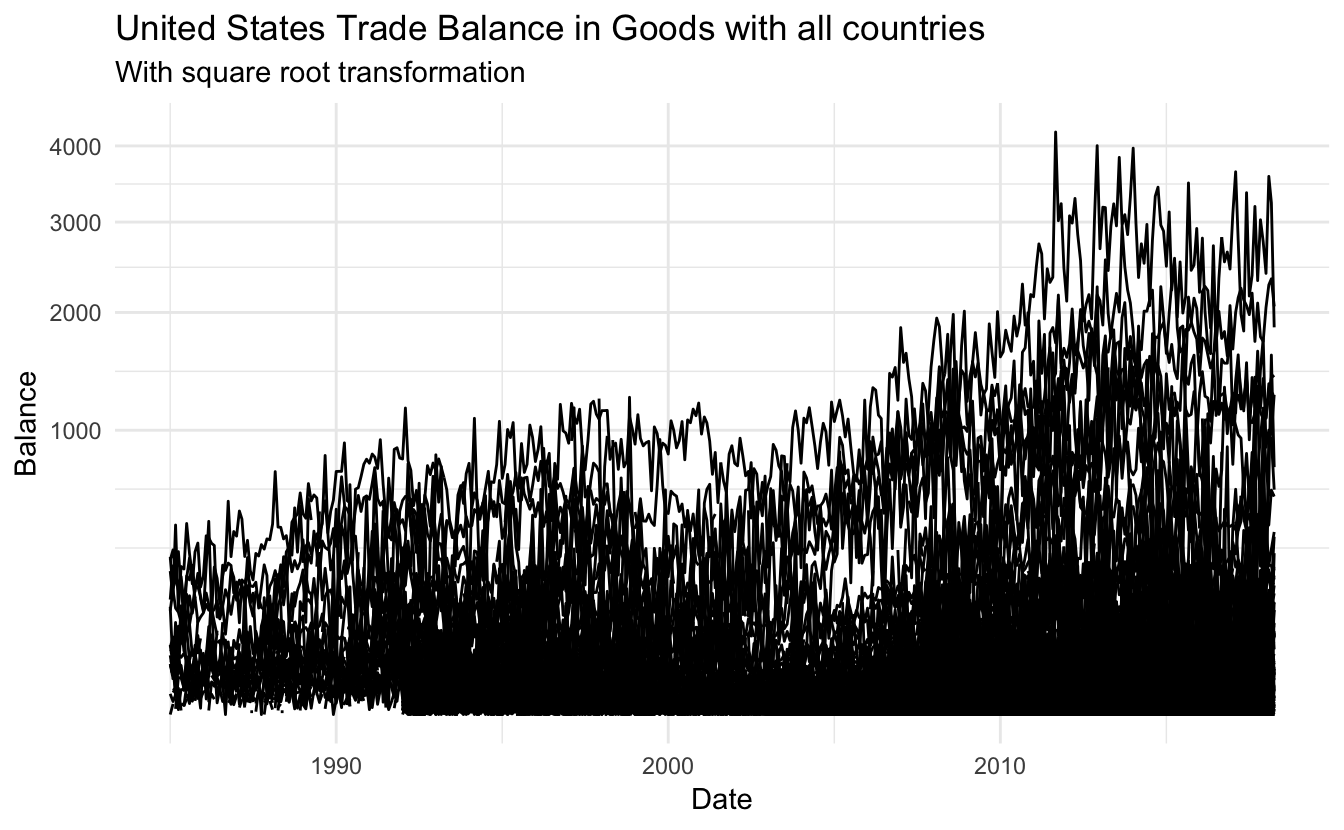Oh no! We lost all the negative values. This happened because the normal square root operation only works with positive numbers. We fix this by using the signed square root which apply the square root to both the positive and negative as if they were positive and then signs them accordingly. for this we create a new transformation with the `scales` package.

``````S_sqrt <- function(x) sign(x) * sqrt(abs(x))
IS_sqrt <- function(x) x ^ 2 * sign(x)
S_sqrt_trans <- function() scales::trans_new("S_sqrt", S_sqrt, IS_sqrt)

ggplot(aes(Date, Balance, group = Country)) +
geom_line() +
coord_trans(y = "S_sqrt") +
labs(title = "United States Trade Balance in Goods with all countries",
subtitle = "With signed square root transformation")``````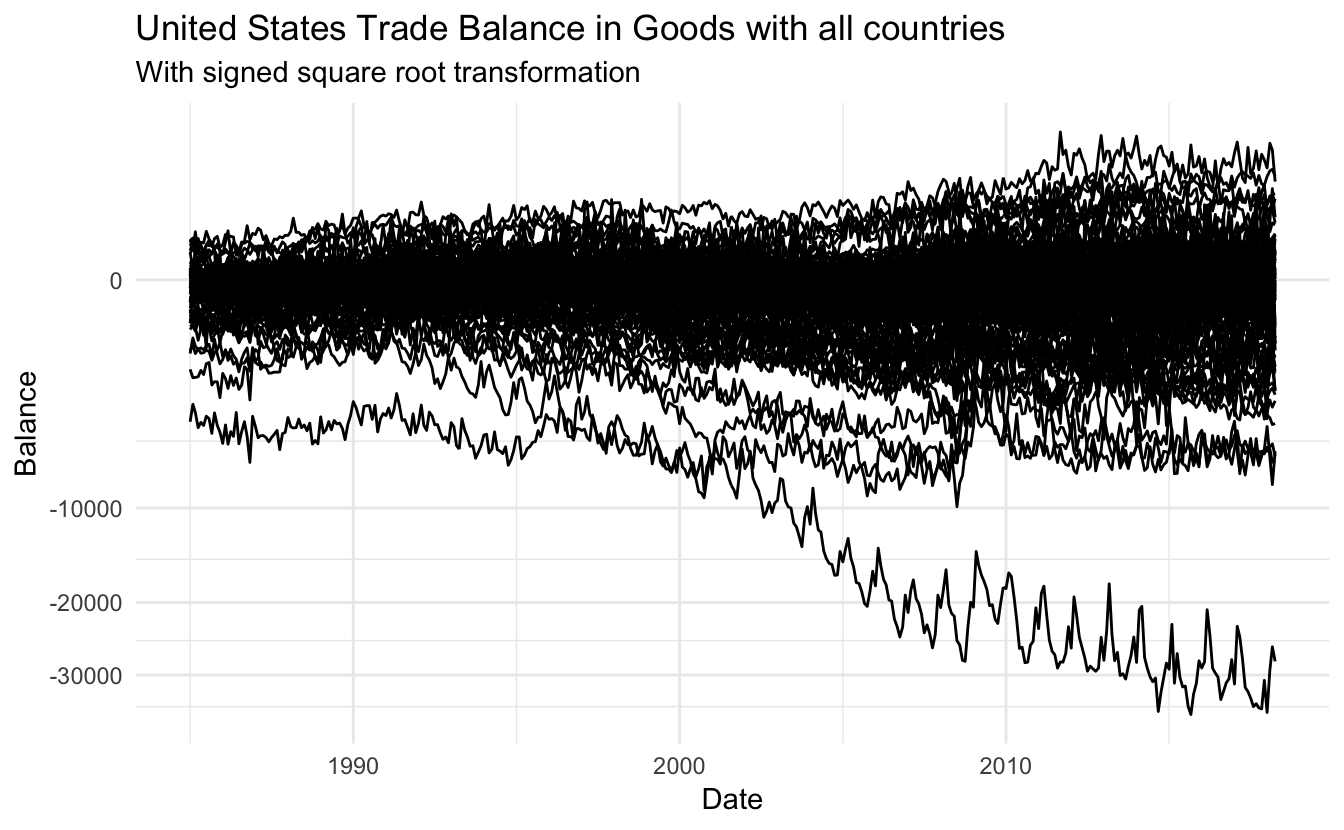Much better! We will fix the the breaks a little bit too.

``````trade_data %>%
ggplot(aes(Date, Balance, group = Country)) +
geom_line() +
coord_trans(y = "S_sqrt") +
scale_y_continuous(breaks = c(0, -1750, -7500, -17000, -30000, 0, 1750, 7500),
minor_breaks = NULL) +
labs(title = "United States Trade Balance in Goods with all countries",
subtitle = "With signed square root transformation")``````Now lets solve the problem with over plotting, a standard trick is to introduce transparency, this is done using the `alpha` aesthetic. Lets start with `0.5` alpha.

``````trade_data %>%
ggplot(aes(Date, Balance, group = Country)) +
geom_line(alpha = 0.5) +
coord_trans(y = "S_sqrt") +
scale_y_continuous(breaks = c(0, -1750, -7500, -17000, -30000, 0, 1750, 7500),
minor_breaks = NULL) +
labs(title = "United States Trade Balance in Goods with all countries",
subtitle = "With signed square root transformation")``````slightly better but not good enough, lets try `0.2`

``````trade_data %>%
ggplot(aes(Date, Balance, group = Country)) +
geom_line(alpha = 0.2) +
coord_trans(y = "S_sqrt") +
scale_y_continuous(breaks = c(0, -1750, -7500, -17000, -30000, 0, 1750, 7500),
minor_breaks = NULL) +
labs(title = "United States Trade Balance in Goods with all countries",
subtitle = "With signed square root transformation")``````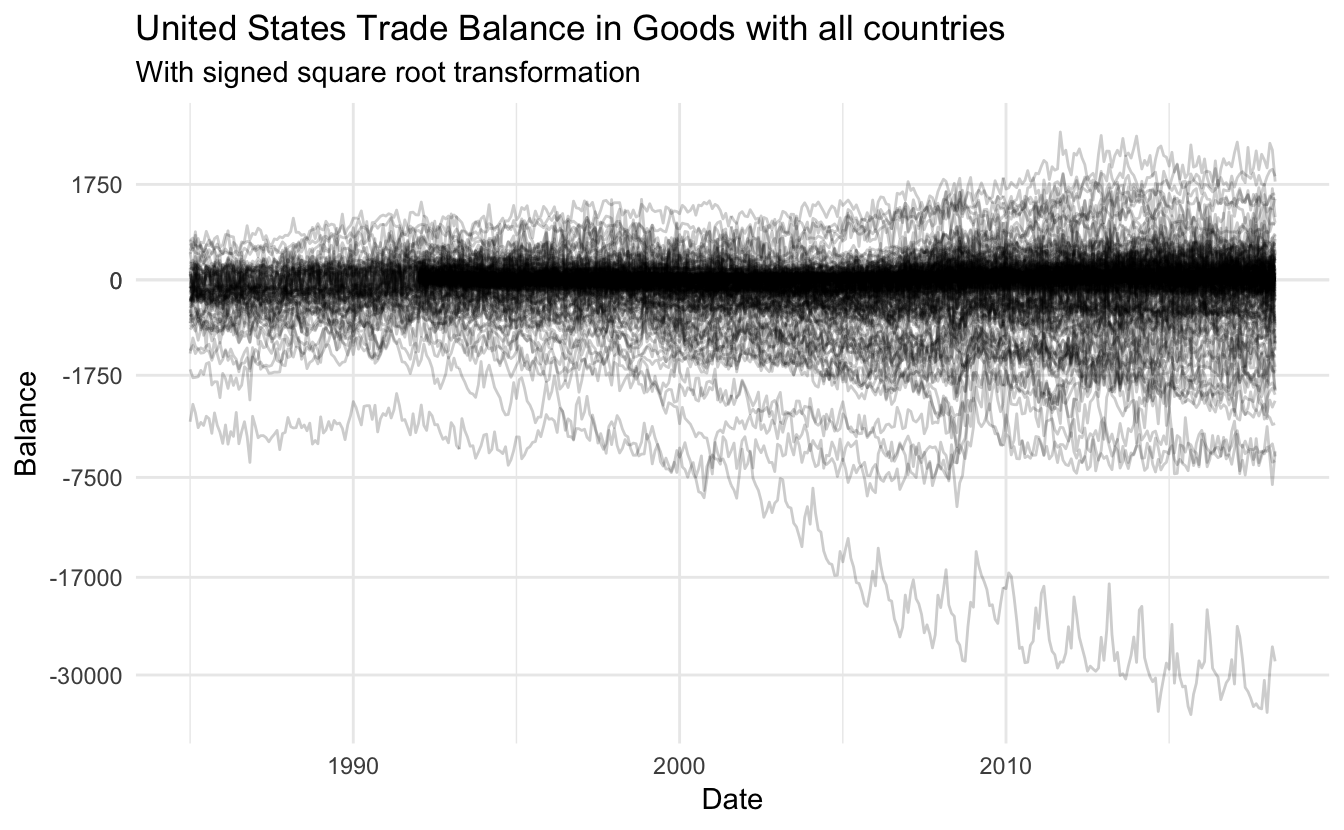much better! Another thing we could do if coloring depending on the the continent.

``````trade_data %>%
ggplot(aes(Date, Balance, group = Country, color = continent)) +
geom_line(alpha = 0.2) +
coord_trans(y = "S_sqrt") +
scale_y_continuous(breaks = c(0, -1750, -7500, -17000, -30000, 0, 1750, 7500),
minor_breaks = NULL) +
labs(title = "United States Trade Balance in Goods with all countries",
subtitle = "With signed square root transformation")``````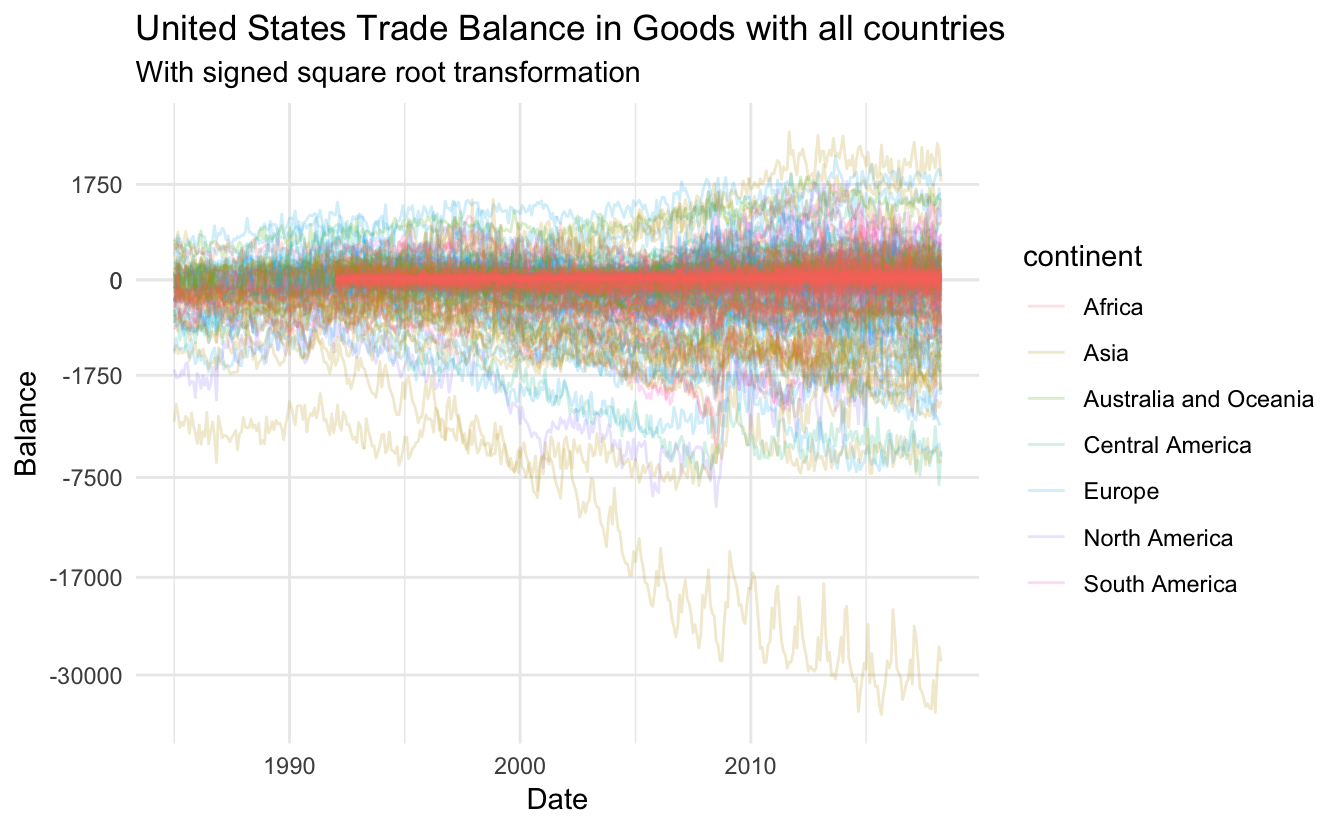This is quite messy, however we notice that the data for the African countries don’t cover the same range as the other countries. Lets see if there are some overall trends within each continent.

``````trade_data %>%
ggplot(aes(Date, Balance, group = Country)) +
geom_line(alpha = 0.2) +
geom_smooth(aes(Date, Balance, group = continent, color = continent), se = FALSE) +
coord_trans(y = "S_sqrt") +
scale_y_continuous(breaks = c(0, -1750, -7500, -17000, -30000, 0, 1750, 7500),
minor_breaks = NULL) +
labs(title = "United States Trade Balance in Goods with all countries",
subtitle = "With signed square root transformation")``````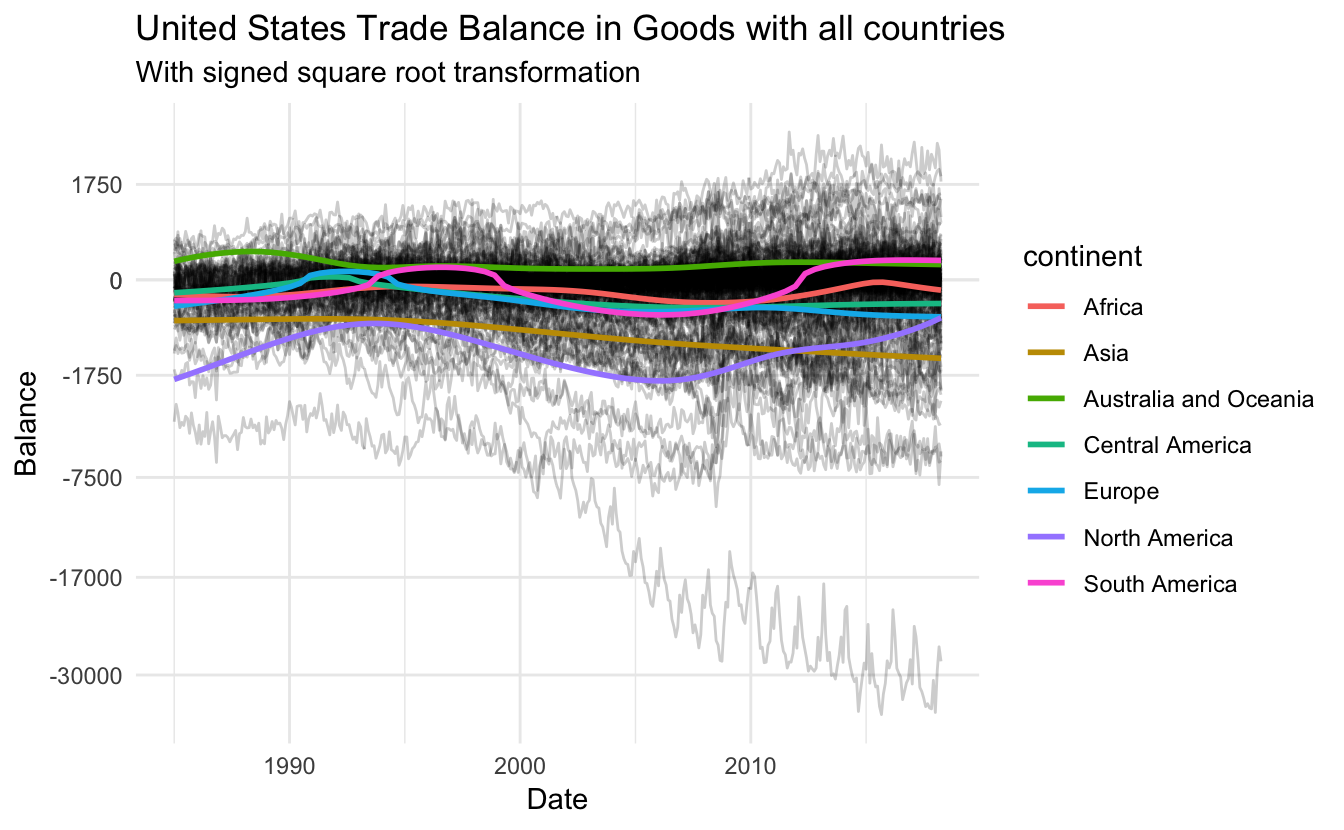This gives some more tangible information. There is a upwards trend within North America for the last 10 years, where Asia have had a slow decline since the beginning of the data collection.

Next lets see what happens when you facet depending on continent

``````trade_data %>%
ggplot(aes(Date, Balance, group = Country)) +
facet_wrap(~ continent) +
geom_line(alpha = 0.2) +
coord_trans(y = "S_sqrt") +
scale_y_continuous(breaks = c(0, -1750, -7500, -17000, -30000, 0, 1750, 7500),
minor_breaks = NULL) +
labs(title = "United States Trade Balance in Goods with all countries",
subtitle = "With signed square root transformation faceted depending on continent")``````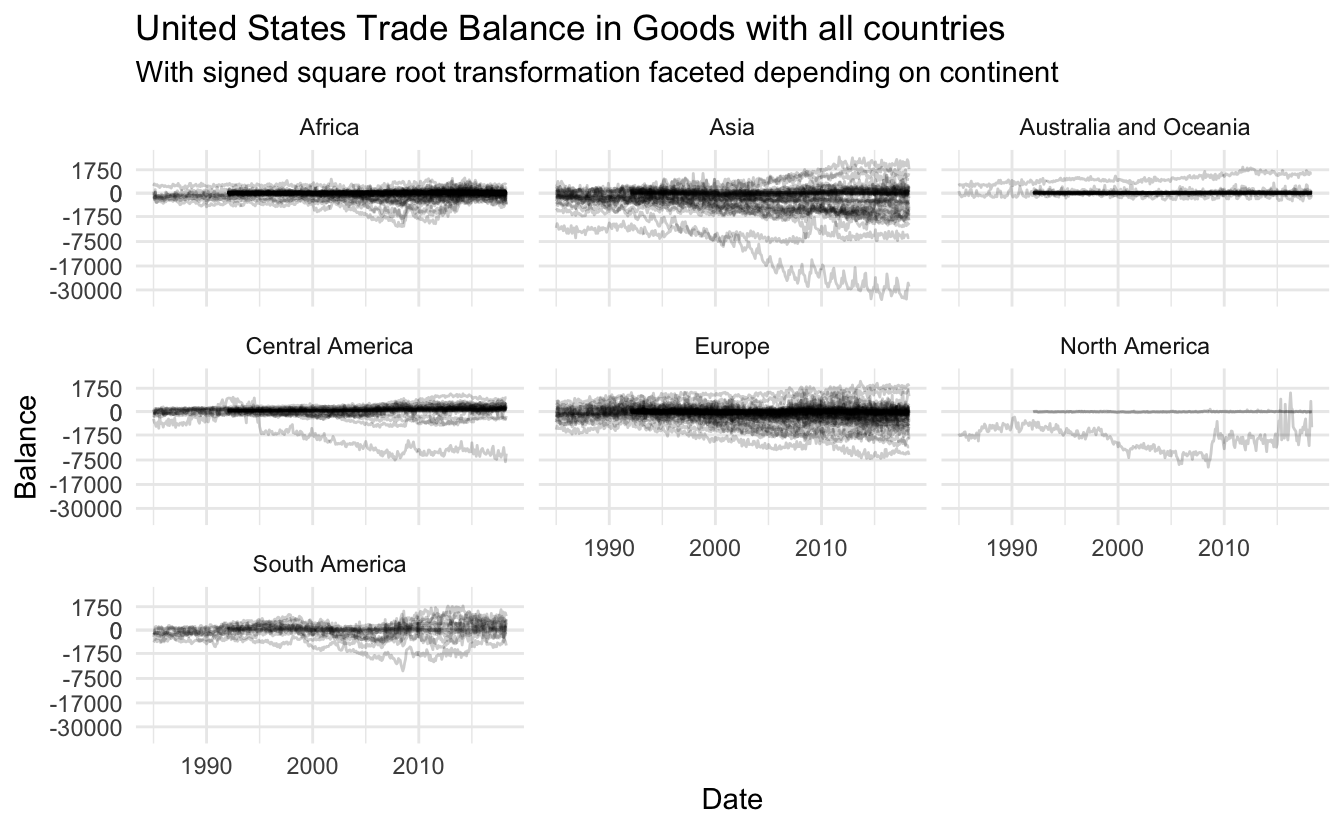These look really nice, lets free up the scale on the y axis within each facet such that we can differentiate the lines better, on top of that lets re introduce the colors.

``````trade_data %>%
ggplot(aes(Date, Balance, group = Country, color = continent)) +
facet_wrap(~ continent, scales = "free_y") +
geom_line(alpha = 0.2) +
coord_trans(y = "S_sqrt") +
scale_y_continuous(breaks = c(0, -1750, -7500, -17000, -30000, 0, 1750, 7500),
minor_breaks = NULL) +
labs(title = "United States Trade Balance in Goods with all countries",
subtitle = "With signed square root transformation faceted depending on continent")``````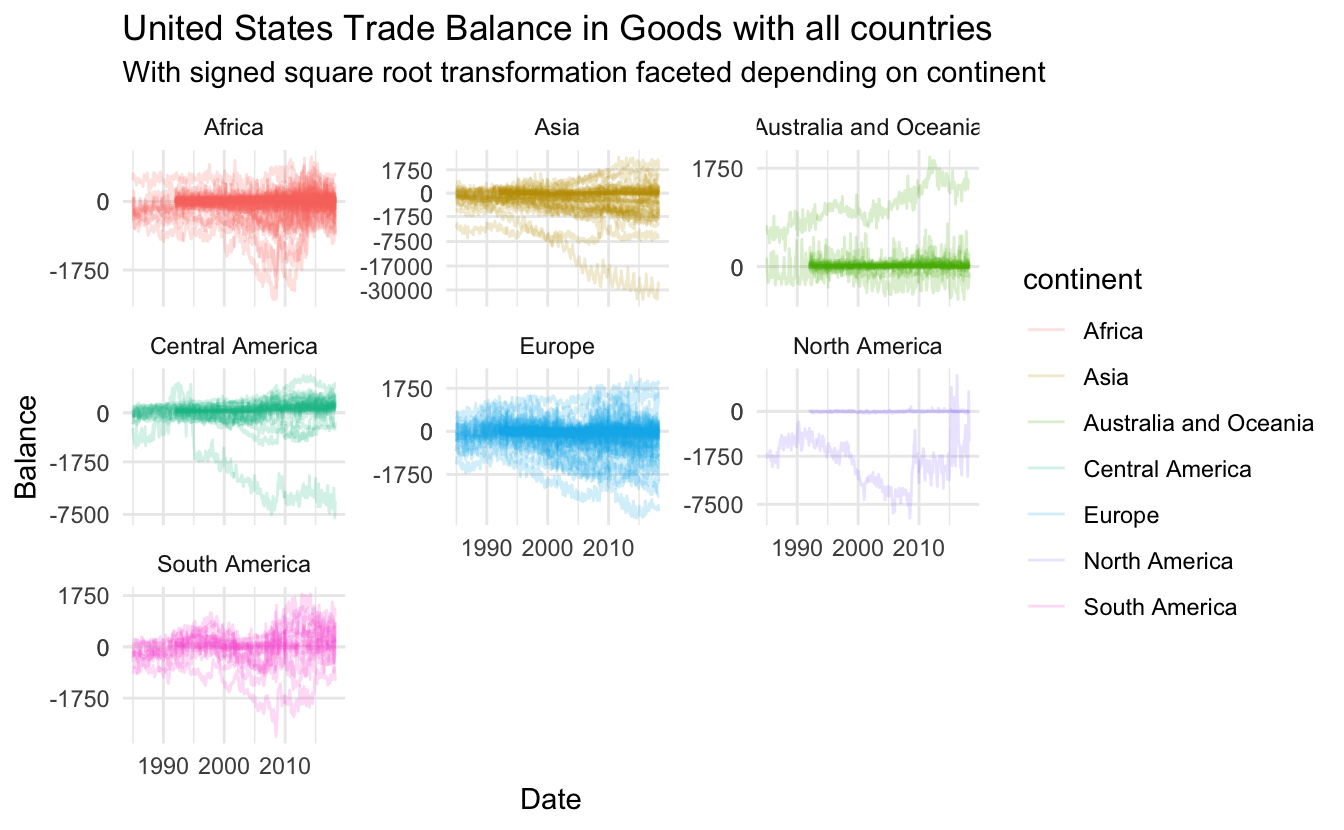lets remove the color legend as the information is already present in the facet labels.

``````trade_data %>%
ggplot(aes(Date, Balance, group = Country, color = continent)) +
facet_wrap(~ continent, scales = "free_y") +
geom_line(alpha = 0.2) +
coord_trans(y = "S_sqrt") +
scale_y_continuous(breaks = c(0, -1750, -7500, -17000, -30000, 0, 1750, 7500),
minor_breaks = NULL) +
labs(title = "United States Trade Balance in Goods with all countries",
subtitle = "With signed square root transformation faceted depending on continent") +
guides(color = "none")``````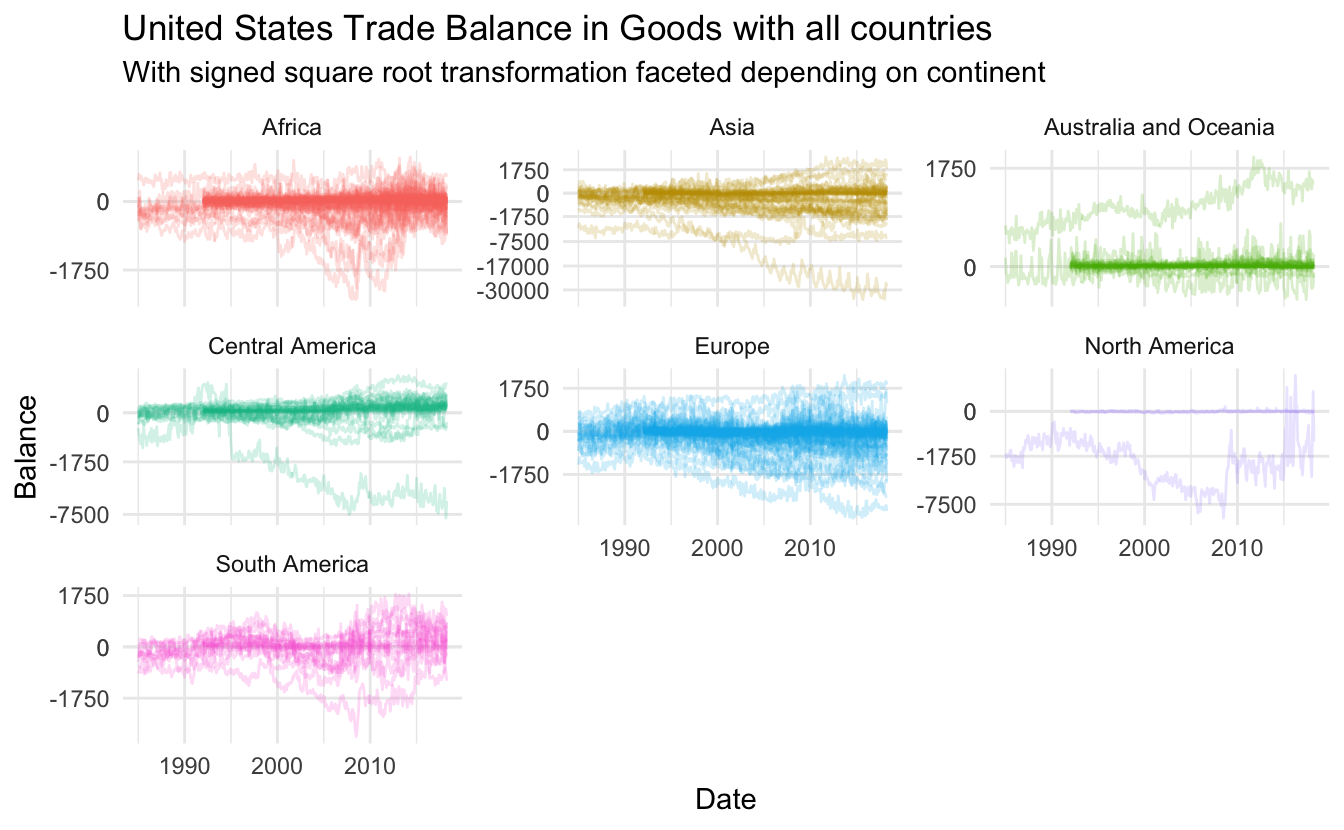Lastly lets overlay the smooth continent average

``````trade_data %>%
ggplot(aes(Date, Balance, group = Country, color = continent)) +
facet_wrap(~ continent, scales = "free_y") +
geom_line(alpha = 0.2) +
geom_smooth(aes(group = continent), color = "grey40", se = FALSE) +
coord_trans(y = "S_sqrt") +
scale_y_continuous(breaks = c(0, -1750, -7500, -17000, -30000, 0, 1750, 7500),
minor_breaks = NULL) +
labs(title = "United States Trade Balance in Goods with all countries",
subtitle = "With signed square root transformation faceted depending on continent") +
guides(color = "none")``````
``## `geom_smooth()` using method = 'gam' and formula 'y ~ s(x, bs = "cs")'``Unfortunately doesn’t add too much information so lets remove it again. Lastly lets update the the labels to reflect the the unit.

``````trade_data %>%
ggplot(aes(Date, Balance, group = Country, color = continent)) +
facet_wrap(~ continent, scales = "free_y") +
geom_line(alpha = 0.2) +
coord_trans(y = "S_sqrt") +
scale_y_continuous(breaks = c(0, -1750, -7500, -17000, -30000, 0, 1750, 7500),
minor_breaks = NULL) +
labs(title = "United States Trade Balance in Goods with all countries",
subtitle = "With signed square root transformation faceted depending on continent",
y = "Balance (in millions of U.S. dollars on a nominal basis)") +
guides(color = "none")``````